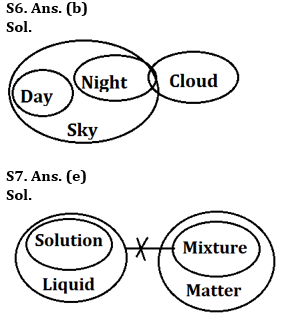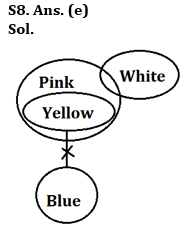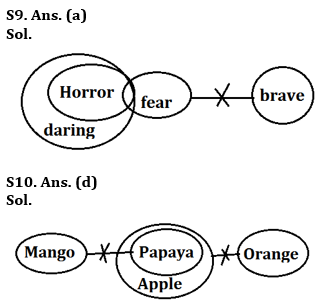Latest Banking jobs   »   IBPS reasoning

# Reasoning Quiz For IBPS PO Prelims 2023-12th September

Directions (1-5): In these questions, relationship between different elements is show in the statements. The statements are followed by conclusions. Study the conclusions based on the given statements and select the appropriate answer:
(a) If only conclusion I follows
(b) If only conclusion II follows
(c) If either conclusion I or II follows
(d) If neither conclusion I nor II follows
(e) If both conclusions I and II follow

Q1. Statements: U > I > X < M ≤ P < L = E ≥ R < T
Conclusion I: U > M
II: E > M

Q2. Statements: W > R = T > Y < M < N ≤ X = D > F
Conclusion I: D ≥ N
II: W > Y

Q3. Statements: S ≥ C > M ≤ O > L = P > R < T > H
Conclusion I: S > L
II: O > T

Q4. Statements: X < M = U ≥ L > J = K > F < G ≤ R ≥ Y
Conclusion I: L ≤ R
II: R > L

Q5. Statements: Z > W > T > D ≤ M < L ≥ I > O ≥ P = Q
Conclusion I: M > Q
II: D < L

Directions (6-10): In each question below are given some statements followed by some conclusions. You have to take the given statements to be true even if they seem to be at variance with commonly known facts. Read all the conclusions and then decide which of the given conclusions logically follows the given statements disregarding commonly known facts. Give answer-
(a) If only conclusion I follow
(b) If only conclusion II follows
(c) If either conclusion I or II follows
(d) If neither conclusion I nor II follows
(e) If both conclusions I and II follow

Q6. Statements:
Some night are cloud
All night are sky
All day are sky
Conclusions:
I. Some night are not day
II. All day being cloud is a possibility

Q7. Statements:
All solution are liquid
No liquid is a mixture
All mixture are matter
Conclusions:
I. All solution being matter is a possibility
II. No solution is a mixtures

Q8. Statements:
Some pink are white
All yellow are pink
No yellow is a blue
Conclusion:
I. Some white, if they are yellow, are also pink
II. All blue being pink is a possibility

Q9. Statements:
Some horror are fear
All horror are daring
No fear is brave
Conclusions:
I. Some horror being brave is a possibility
II. No horror is brave

Q10. Statements:
No mango is papaya
All papaya are apple
No apple is orange
Conclusions:
I. All orange being papaya is a possibility
II. Some mango are not orange

Solutions

S1. Ans(b)
Sol. I: U>M(False)
II: E>M(True)

S2. Ans(e)
Sol. I: D≥N(True)
II: W>Y(True)

S3. Ans(d)
Sol. I: S>L(False)
II: O>T(False)

S4. Ans(d)
Sol. I: L≤R(False)
II: R>L(False)

S5. Ans(b)
Sol. I: M>Q(False)
II: D<L(True)## FAQs

### When will the IBPS PO prelims 2023 be conducted?

IBPS PO Prelims will be conducted on 23, 30 September, and 1 October 2023.

#### Congratulations!Union Budget 2023-24: Free PDF